Question

About 7% of the population has a particular genetic mutation. 800 people are randomly selected. Find the standard deviation for the number of people with the genetic mutation in such groups of 800.

1.The standard deviation is of 7.22 people.

Step-by-step explanation:

For each person, there are only two possible outcomes. Either they have the mutation, or they do not. The probability of a person having the mutation is independent of any other person, which means that the binomial probability distribution is used to solve this question.

Binomial probability distribution

Probability of exactly x successes on n repeated trials, with p probability.

The expected value of the binomial distribution is: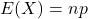The standard deviation of the binomial distribution is: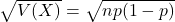About 7% of the population has a particular genetic mutation.

This means that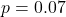800 people are randomly selected.

This means that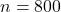Find the standard deviation for the number of people with the genetic mutation in such groups of 800.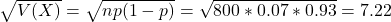The standard deviation is of 7.22 people.